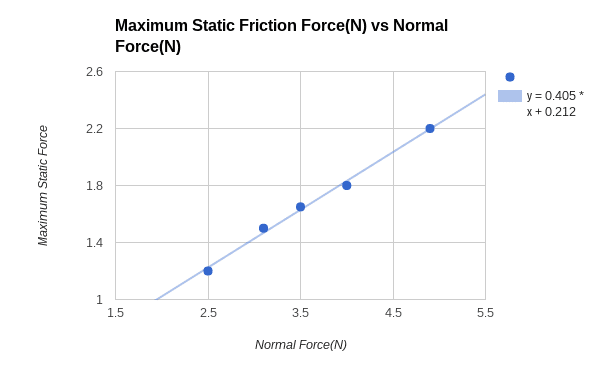# How to find the Coefficient of friction from Fn and Ff Graph

## Homework Statement

I have a Force Normal vs Force Friction Graph and I wan to find the coefficient of friction from the graph.

## Homework Equations## The Attempt at a Solution

I have tried the formula ff= uFN but my teacher is looking for me to do it using the graph

## Answers and Replies

kuruman
Science Advisor
Homework Helper
Gold Member
##F^{max}_s=\mu_s~F_N## is of the form y = slope * x.

•CWatters
CWatters
Science Advisor
Homework Helper
Gold Member
+1

Look up the general equation for a straight line. Usually expressed as

Y=mx + c

m is the slope
c is the vertical offset (point where the line intercepts the y axis).